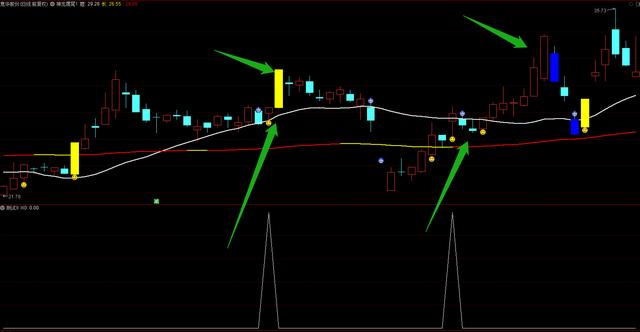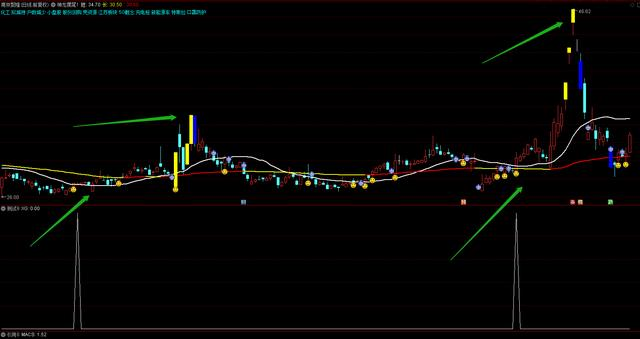# 股价涨停回调，中线一阳指位置出信号向上趋势明显# 具体的公式源码为：

A3:=SMA((CLOSE-A1)/(A2-A1)*100,5,1);

AA:=A3;

BB:=A4;

A5:=SMA(MAX(CLOSE-REF(CLOSE,1),0),5,1)/SMA(ABS(CLOSE-REF(CLOSE,1)),5,1)*100;

XG: CROSS(CC,BB)AND CC>=REF(CC,1) AND BB<=REF(BB,3)AND CC>=49.5 AND MA(C,3)>=REF(MA(C,3),1)AND MA(C,7)>=REF(MA(C,7),1) AND MA(C,60)>REF(MA(C,60),3);# 具体的源码解析为：

A2赋值:13日内最高价的最高值

A3赋值:(收盘价-A1)/(A2-A1)*100的5日[1日权重]移动平均

A5赋值:收盘价-1日前的收盘价和0的较大值的5日[1日权重]移动平均/收盘价-1日前的收盘价的绝对值的5日[1日权重]移动平均*100